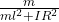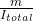## Consider the case in which the clay is launched along Path Y. The sphere of clay is launched with an initial velocity of v0 and collides wit

Question

Consider the case in which the clay is launched along Path Y. The sphere of clay is launched with an initial velocity of v0 and collides with the rod at a distance of l from the pivot. The length of the rod is L. The rotational inertia of the rod about the joint is IR, and the mass of the clay is mc. The clay is considered a point mass. What is the angular speed f of the clay-rod system immediately after the collision

in progress 0
2 months 2021-07-23T12:11:09+00:00 1 Answers 2 views 0

w =v l

Explanation:

Let’s form a system formed by the clay sphere and the rod, in this case the angular momentum is conserved

initial instant. Before the crash

L₀ = m v l

Final moment. After the collision with the clay stuck to the rod

L_f = I_{total} w

angular momentum is conserved

L₀ = L_f

m v l = I_total w

w =v l

the total moment of inertia is the sum of the moments of inertia of the two bodies

the moment of inertia of the rod is

I_rod = I R²

I_total = m l² + IR²

we substitute

w =v l